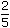# Aptitude - Ratio and Proportion

## Why Aptitude Ratio and Proportion?

In this section you can learn and practice Aptitude Questions based on "Ratio and Proportion" and improve your skills in order to face the interview, competitive examination and various entrance test (CAT, GATE, GRE, MAT, Bank Exam, Railway Exam etc.) with full confidence.

## Where can I get Aptitude Ratio and Proportion questions and answers with explanation?

IndiaBIX provides you lots of fully solved Aptitude (Ratio and Proportion) questions and answers with Explanation. Solved examples with detailed answer description, explanation are given and it would be easy to understand. All students, freshers can download Aptitude Ratio and Proportion quiz questions with answers as PDF files and eBooks.

## Where can I get Aptitude Ratio and Proportion Interview Questions and Answers (objective type, multiple choice)?

Here you can find objective type Aptitude Ratio and Proportion questions and answers for interview and entrance examination. Multiple choice and true or false type questions are also provided.

## How to solve Aptitude Ratio and Proportion problems?

You can easily solve all kind of Aptitude questions based on Ratio and Proportion by practicing the objective type exercises given below, also get shortcut methods to solve Aptitude Ratio and Proportion problems.

### Exercise :: Ratio and Proportion - General Questions

1.

A and B together have Rs. 1210. Ifof A's amount is equal toof B's amount, how much amount does B have?

 A. Rs. 460 B. Rs. 484 C. Rs. 550 D. Rs. 664

Explanation:

 4 A = 2 B 15 5A =2 x 15B 5 4A = 3 B 2A = 3 B 2A : B = 3 : 2.B's share = Rs.1210 x 2= Rs. 484. 5

2.

Two numbers are respectively 20% and 50% more than a third number. The ratio of the two numbers is:

 A. 2 : 5 B. 3 : 5 C. 4 : 5 D. 6 : 7

Explanation:

Let the third number be x.

 Then, first number = 120% of x = 120x = 6x 100 5

 Second number = 150% of x = 150x = 3x 100 2Ratio of first two numbers =6x : 3x= 12x : 15x = 4 : 5. 5 2

3.

A sum of money is to be distributed among A, B, C, D in the proportion of 5 : 2 : 4 : 3. If C gets Rs. 1000 more than D, what is B's share?

 A. Rs. 500 B. Rs. 1500 C. Rs. 2000 D. None of these

Explanation:

Let the shares of A, B, C and D be Rs. 5x, Rs. 2x, Rs. 4x and Rs. 3x respectively.

Then, 4x - 3x = 1000x = 1000.B's share = Rs. 2x = Rs. (2 x 1000) = Rs. 2000.

4.

Seats for Mathematics, Physics and Biology in a school are in the ratio 5 : 7 : 8. There is a proposal to increase these seats by 40%, 50% and 75% respectively. What will be the ratio of increased seats?

 A. 2 : 3 : 4 B. 6 : 7 : 8 C. 6 : 8 : 9 D. None of these

Explanation:

Originally, let the number of seats for Mathematics, Physics and Biology be 5x, 7x and 8x respectively.

Number of increased seats are (140% of 5x), (150% of 7x) and (175% of 8x).140 x 5x,150 x 7xand175 x 8x100 100 1007x, 21x and 14x. 2The required ratio = 7x : 21x : 14x 214x : 21x : 28x2 : 3 : 4.

5.

In a mixture 60 litres, the ratio of milk and water 2 : 1. If this ratio is to be 1 : 2, then the quantity of water to be further added is:

 A. 20 litres B. 30 litres C. 40 litres D. 60 litres

Explanation:

 Quantity of milk =60 x 2litres = 40 litres. 3

Quantity of water in it = (60- 40) litres = 20 litres.

New ratio = 1 : 2

Let quantity of water to be added further be x litres.

 Then, milk : water =40. 20 + x

 Now,40= 1 20 + x 220 + x = 80x = 60.Quantity of water to be added = 60 litres.